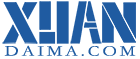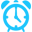# PHP退出循环

php跳出循环只有continue和break两个语句，continue语句只能终止本次循环，并进入下一次循环中。

## 跳出循环案例：

```<?php
\$a = 1;
while (true) { // 这里看上去这个循环会一直执行
if (\$a==2) {// 2跳过不显示
\$a++;
continue;          //跳出本次循环，本次循环体中的代码不在执行，故本次循环结尾的\$a++不会在执行。即控制程序放弃本次循环continue

} else if (\$a==5) {// 但到这里\$a=5就跳出循循环了
break;       //break直接结束循环体，语法break N 可直接定义跳出几层循环
} else {
echo \$a . '<br>';
}
\$a++;
}
?>```

## php跳出foreach循环

```<?php
\$a = array("0"=>"arr","1"=>"brr","2"=>"crr","3"=>"drr");
foreach (\$a as \$key => \$value) {
if (\$key==1) {
//\$key为数组的标记，如果没有设置第一个从0开始
continue;//到这里,就跳出brr那次循环
}else if (\$key==3) {
break;       //到这里，就是drr就直接结束循环体
} else {
echo \$a[\$key] . '<br>'; //输出第几个元素
}

}```

arr

crr

## php跳出本次循环

```<?php
// \$a=1;
// while(\$a<=10);
// {
//   if (\$a==5) {
//        continue;//退出本次循环
//   }
//   echo \$a;
//   \$a++;
// }
//注销部分是死循环

for(\$b=1;\$b<=10;\$b++){
if (\$b==5) {
continue;//退出本次循环
}
echo \$b;
}
?>```

### 结果：

1

2

3

4

6

7

8

9

10while和do...while在执行continue的会陷入死循环

## PHP跳出for循环

```<?php

for(\$a=1;\$a<=3;\$a++){
for(\$b=1;\$b<=3;\$b++){
for(\$c=1;\$c<=3;\$c++){
if (\$c==2) {
//在\$c等于2的时退出\$b那for
break 2; //break 后面跟数字代表退出层数循环2代表由内到外数
//continue 同理，数字代表忽略那层本次循环由，内到外数

}
echo "第".\$a."号,第".\$b."层,第".\$c."次"."</br>";

}

}
}
?>```